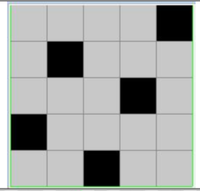Related Articles

# How to change imshow aspect ratio in Matplotlib?

• Last Updated : 03 Jan, 2021

Aspect ratio is the ratio of height to width of the image we want to display. Matplotlib provides us the feature of modifying the aspect ratio of our image by specifying the value for the optional aspect ratio attribute for our image plot.

Syntax: pyplot.imshow(image, aspect=’value’)

We can replace the value for the aspect ratio with ‘equal’, ‘auto’, or any float value representing the desired aspect ratio. For this article, we have used the below image to demonstrate the changes in aspect ratio using Matplotlib.Original Image

Example:

## Python3

 `import` `matplotlib.pyplot as plt``import` `cv2`` ` `# reading image from directory``im ``=` `cv2.imread(``"C://Users/User/Downloads/chess5.png"``)`` ` `# plotting a figure for showing all ``# images in a single plot``fig ``=` `plt.figure(figsize``=``(``4``, ``4``))`` ` `# plotting each matplot image with``# different aspect ratio parameter values``# in a seperate subplot``ax1 ``=` `fig.add_subplot(``2``, ``2``, ``1``)``ax1.set_xlabel(``'Original'``)`` ` `# plot the initial image as the first image``plt.imshow(im)`` ` `ax2 ``=` `fig.add_subplot(``2``, ``2``, ``2``)``ax2.set_xlabel(``'Aspect Ratio : Auto'``)`` ` `# plot the image with "auto" aspect ratio``# as the second image``plt.imshow(im, aspect``=``'auto'``)`` ` `ax3 ``=` `fig.add_subplot(``2``, ``2``, ``3``)``ax3.set_xlabel(``'Aspect Ratio : 0.5'``)`` ` `# plot the image with "0.5" aspect ratio ``# as the third image``plt.imshow(im, aspect``=``'0.5'``)`` ` `ax4 ``=` `fig.add_subplot(``2``, ``2``, ``4``)``ax4.set_xlabel(``'Aspect Ratio : 1.5'``)`` ` `# plot the image with "1.5" aspect ratio``# as the fourth image``plt.imshow(im, aspect``=``'1.5'``)`` ` `# display the plot``plt.show()`

Output:Attention geek! Strengthen your foundations with the Python Programming Foundation Course and learn the basics.

To begin with, your interview preparations Enhance your Data Structures concepts with the Python DS Course. And to begin with your Machine Learning Journey, join the Machine Learning – Basic Level Course

My Personal Notes arrow_drop_up